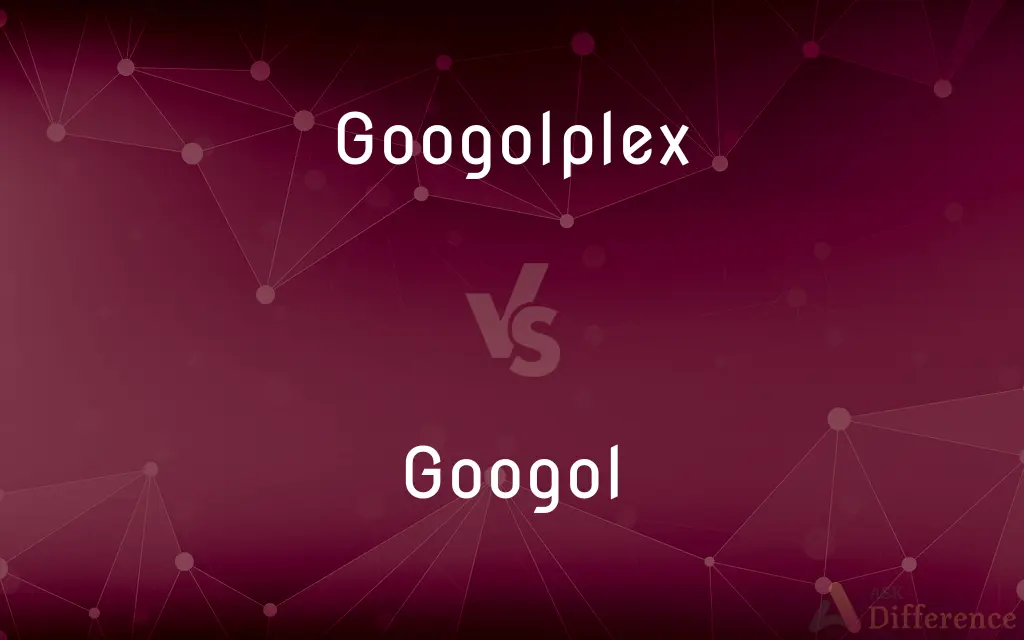# Googolplex vs. Googol — What's the Difference?## Definitions

#### Googolplex➦

A googolplex is the number 10googol, or equivalently, 10(10100). Written out in ordinary decimal notation, it is 1 followed by 10100 zeroes; that is, a 1 followed by a googol zeroes.

#### Googol➦

A googol is the large number 10100. In decimal notation, it is written as the digit 1 followed by one hundred zeroes: 10,​000,​000,​000,​000,​000,​000,​000,​000,​000,​000,​000,​000,​000,​000,​000,​000,​000,​000,​000,​000,​000,​000,​000,​000,​000,​000,​000,​000,​000,​000,​000,​000,​000.

#### Googolplex➦

The number 10 raised to the power googol, written out as the numeral 1 followed by 10100 zeros.

#### Googol➦

The number 10 raised to the power 100 (10100), written out as the numeral 1 followed by 100 zeros.

#### Googolplex➦

The number $10^\left\{10^\left\{100\right\}\right\}$ or $10^\text\left\{googol\right\}$, ten to the power of a googol.

#### Googol➦

The number $10^\left\{100\right\}$, or ten to the power of a hundred. Equivalent to ten duotrigintillion (short scale), or ten sedecilliard (long scale).

#### Googolplex➦

(figuratively) A countable number

#### Googol➦

A cardinal number represented as 1 followed by 100 zeros (ten raised to the power of a hundred)

#### Googolplex➦

An infinitesimally small portion of land, defined for legal purposes.

#### Googolplex➦

A cardinal number represented as 1 followed by a googol of zeros (ten raised to the power of a googol)